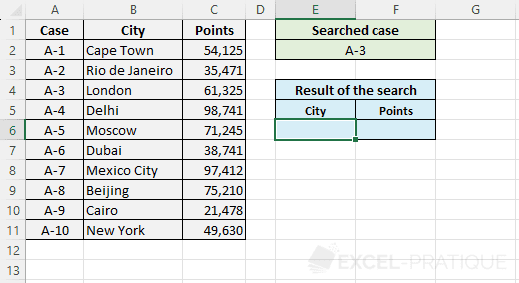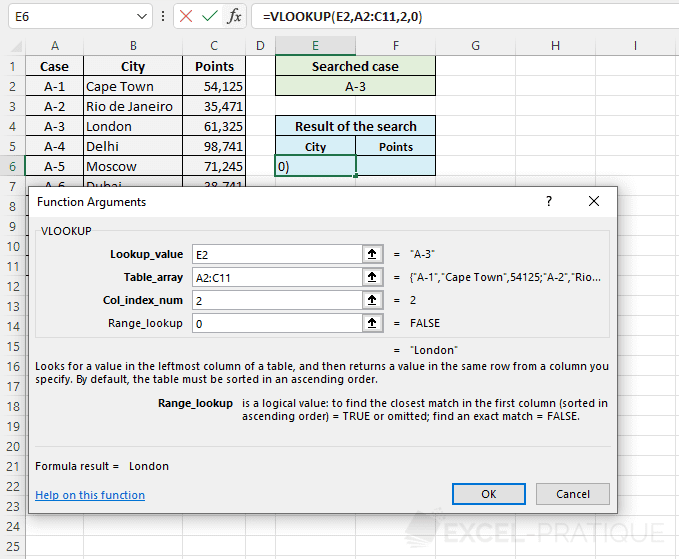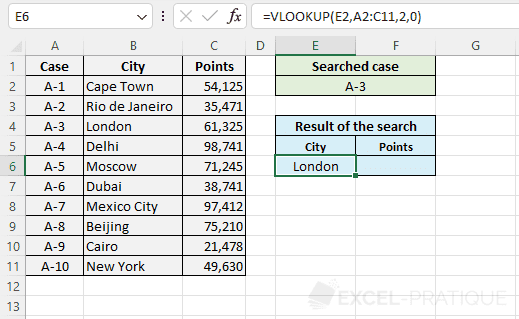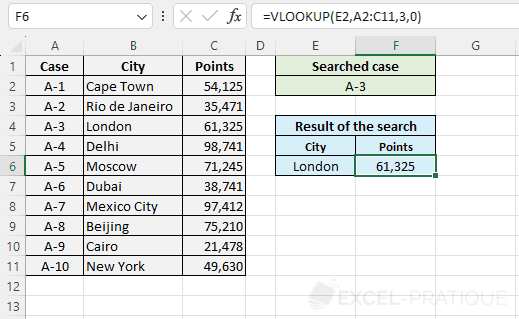# Excel Function: VLOOKUP

The Excel function VLOOKUP searches for a value in the first column of a table and then returns the value of a cell that is on the same row as the searched value.

Usage:

`=VLOOKUP(lookup_value, table_array, col_index_num, range_lookup)`

## Example of Use

The goal here is to look up information based on the file number. The user should be able to enter the file number in the green part and then see the result of his search in the blue part:Select the VLOOKUP function and enter:

• Lookup_value: the value to search for in the first column of the table (here, the file number)
• Table_array: the range of cells containing the table data
• Col_index_num: the column number in the table that contains the result to return (here, column 2 for the city)
• Range_lookup: FALSE or 0 to search for the exact value of Lookup_value (when in doubt, enter 0 to avoid surprises), TRUE or 1 (or leave empty) to search for the closest value to Lookup_value
``=VLOOKUP(E2,A2:C11,2,0)``The name of the city is then displayed:To then display the points, simply copy the formula and change the column number (replace 2 with 3):